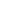Three working states of stepping motor.The characteristics of stepping drive are determined by the respective characteristics of driving circuit, mechanical structure and stepping motor.

The working state of stepping motor can be divided into static state, steady state and transition state.

1. Static

Static state refers to the instantaneous locking state of the rotor, that is, the direct current (pulse frequency f = 0) is applied in the motor control winding, and the rotor is in a locked state. In this state, the phase current of the motor winding is the largest, and the winding is not replaced, so the motor emits uneven heat in the connected phase (not all). Fever is one of the most serious conditions.

The stable synchronization state occurs when the frequency of the control pulse is constant. At this time, the rotor rotates at a constant speed, which can also be considered as periodic fluctuation relative to the synchronous speed. This state can be divided into limit (i.e. under continuous frequency) and non limit.
The maximum pulse frequency Fmax of the motor output shaft under a certain load and the corresponding maximum speed nmax of the rotor are the limit synchronization state. In this state, the rotor actually rotates uniformly without swing. This state can only be achieved under certain startup procedures. When the pulse frequency is higher than Fmax, the rotor is out of synchronization (i.e. out of step).
When the control frequency is less than Fmax, the motor is in a non limit stable state. This state often involves a stable swing (oscillation) of the rotor, which is particularly dangerous in the resonant frequency region.

3. Basic transition state

(1) When the frequency changes from zero to start FQ, the speed of the motor rotor accelerates from zero to start at the limit speed (usually the movement limit frequency is called the no-load maximum dynamic frequency). It is impossible to start the motor without out of step above the maximum mutation frequency FQ (commonly referred to as starting frequency or sensitivity frequency).

(2) When the control pulse is suddenly interrupted, the motor decelerates sharply from the stable synchronization state to parking locking. The frequency in this stable synchronization state is called the stable limit braking frequency FS. Above this frequency, it is impossible to brake without sliding. Only gradually reducing the frequency and then braking can ensure no impact.

(3) The motor is reversed. When the alternating sequence of winding replacement is suddenly changed, the rotor changes from a stable synchronous turntable in one rotation direction to another stable synchronous state in the opposite direction. There is also a limit inversion frequency ft. Above this frequency, the rotor will be out of step, that is, there is an error with the given program information.
Starting, braking and reversing can be performed under various (non-zero) initial conditions (angles) θ And speed η） Implemented under.
However, the initial conditions strongly affect the specific values of FQ, FS and FT, so the drag calculation is difficult. Therefore, there should be initial conditions when studying the frequency characteristics of the motor, and the initial conditions of hard opening rate should be included in the design program. Similarly, when selecting the motor, we should also know the experimental conditions of these frequency characteristics and the data acquisition (including the initial conditions) in detail.wechat QR codeChat with robot Hermione